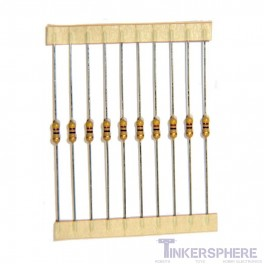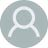Maximize

# Single Value Resistor 10 pack : 1/4W 5% Carbon-Film

Need a specific resistor value for your project? Select the exact resistor value you need! Over 120 resistance values to choose from between 0.33Ω and 4.7MΩ.

Availability: SHIPS TODAY

Last Updated: 10/04/2022

Tinkersphere

\$1.49

This 10 pack of standard 1/4W 5% Carbon-Film Resistors is useful for nearly all electronics projects. Select the values you'd like from the drop-down menu above. Includes 10 resistors of any single value.

Available values:

• 0.33 Ohm
• 1 Ohm
• 1.5 Ohm
• 2.2 Ohm
• 2.7 Ohm
• 3.3 Ohm
• 3.9 Ohm
• 4.7 Ohm
• 5.1 Ohm
• 6.2 Ohm
• 6.8 Ohm
• 7.5 Ohm
• 8.2 Ohm
• 10 Ohm
• 12 Ohm
• 15 Ohm
• 18 Ohm
• 20 Ohm
• 22 Ohm
• 24 Ohm
• 27 Ohm
• 30 Ohm
• 33 Ohm
• 36 Ohm
• 39 Ohm
• 43 Ohm
• 47 Ohm
• 51 Ohm
• 56 Ohm
• 62 Ohm
• 68 Ohm
• 75 Ohm
• 82 Ohm
• 91 Ohm
• 100 Ohm
• 120 Ohm
• 150 Ohm
• 180 Ohm
• 200 Ohm
• 220 Ohm
• 240 Ohm
• 270 Ohm
• 300 Ohm
• 330 Ohm
• 360 Ohm
• 390 Ohm
• 430 Ohm
• 470 Ohm
• 510 Ohm
• 560 Ohm
• 620 Ohm
• 680 Ohm
• 750 Ohm
• 820 Ohm
• 830 Ohm
• 910 Ohm
• 1K Ohm
• 1.2K Ohm
• 1.5K Ohm
• 1.8K Ohm
• 2K Ohm
• 2.2K Ohm
• 2.4K Ohm
• 2.7K Ohm
• 3K Ohm
• 3.3K Ohm
• 3.6K Ohm
• 3.9K Ohm
• 4.3K Ohm
• 4.7K Ohm
• 5.1K Ohm
• 5.6K Ohm
• 6.2K Ohm
• 6.8K Ohm
• 7.5K Ohm
• 8.2K Ohm
• 9.1K Ohm
• 10K Ohm
• 12K Ohm
• 15K Ohm
• 18K Ohm
• 20K Ohm
• 22K Ohm
• 24K Ohm
• 27K Ohm
• 30K Ohm
• 33K Ohm
• 36K Ohm
• 39K Ohm
• 43K Ohm
• 47K Ohm
• 51K Ohm
• 56K Ohm
• 62K Ohm
• 68K Ohm
• 75K Ohm
• 82K Ohm
• 91K Ohm
• 100K Ohm
• 120K Ohm
• 150K Ohm
• 180K Ohm
• 200K Ohm
• 220K Ohm
• 240K Ohm
• 270K Ohm
• 300K Ohm
• 330K Ohm
• 360K Ohm
• 390K Ohm
• 430K Ohm
• 470K Ohm
• 510K Ohm
• 560K Ohm
• 620K Ohm
• 680K Ohm
• 750K Ohm
• 820K Ohm
• 910K Ohm
• 1M Ohm
• 2.2M Ohm
• 4.7M Ohm
If I order this online, when can I pick it up at the New York store?
On the next business day. If you place your order before midnight, you can pick it up tomorrow anytime after 12pm.Ricardo · 11/20/2019